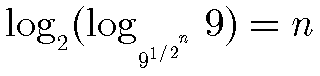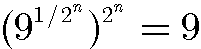# A single formula to express every positive integer with digits 0-9 in which every digit is used only once.Ross Honsberger (Mathematical Gems III, MAA, 1985, p 175) credits Verner Hoggatt Jr. with the following formula,

where there are exactly n square roots. If the fraction in the first base is written as (0 + 1 + 2 + 3 + 4)/5, then all digits enter the formula in their natural order. Simplified the formula appears as,

which is equivalent to,

which, in turn, states the obvious• Funny Arithmetic
• Any Integer with Three 2s
• Representation of numbers with a single 4. The rules and the possibilities
• One 4, a story
• Three 3's
• Representation of numbers with three 4's
• Representation of numbers with three 5's
• Representation of numbers with four 3's
• Representation of numbers with four 4's
• Representation of numbers with four 5's
• A problem of representing 4 by four identical digits
• A problem of representing 6 by three identical digits
• A 9's Fan's Clock
• Make an Identity
• Fun with numbers: place plus/minus signs between the digits
• 24 with One Digit
• Representation of 3 with Three Equal Digits
•